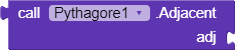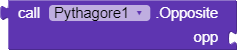# Pythagore - My first extension

An extension for study the theorem of Pythagoreit’s a function calculating the square of the adjacent.it’s a function calculating the square of the opposite.it’s a property returning the value of Opposite or Adjacent.

Here’s the code:5 Likes

A post was merged into an existing topic: Pythagore extension new version

An extension for study the theorem of Pythagoreit’s a function calculating the square of the adjacent.it’s a function calculating the square of the opposite.it’s a property returning the value of Opposite or Adjacent.

Here’s the code:5 Likes

An extension calculating the hypothenuse of a triangle.is a property getting the pythagore function valuesis a function calculating the sum between the square of the adjacent and the square of the opposite.

Here’s the code:

2 Likes

Nice work!
You might want to edit your Extension’s previous topic instead of creating a new topic… Every Extension should have one topic only2 Likes

allright. and thanks.

1 Like

Is this extension same as described hereNice work! However, this can easily achieved by very few blocks. Try making extensions that can’t or hard to be achieved by the default blocks.

your extension is interesting, but you should name your components in english.
and create your own topic for publish it.
But i am interested. I will test it.

The extension I quoted above doesn’t belong to me.I am not the developer of that extension. Also I quoted that topic because both the extension seemed similar to me